# Lineplot and Stacked area chart with R and ggplot2

An original and nice-looking visualization combining a lineplot and a stacked area chart with several customizations to explore the evolution of child labour made with `R` and `ggplot2`. This blogpost guides you through a step-by-step construction of the charts and how to combine them into a single piece of art with the `patchwork` library.

Thanks to them for all the inspiring and insightful visualizations! Thanks also to Tomás Capretto who replicated the chart in R! 🙏🙏

As a teaser, here is the plot we’re gonna try building:It is possible to think it is not going to be too much work to reproduce today’s chart because, at first sight, there’s nothing that looks very fancy. However, it actually contains several subtle customizations that when added all together make the final result look original and beautiful.

This is article uses several plotting libraries apart from the nice `ggplot2` we always use. The first one is `shadowtext`, a library that allows to draw text with shadows. Then, the popular `patchwork`, which is going to make the task of combining `ggplot2` figures extremely easy. And finally, we’re also going to use the `grid` library, the drawing library behind `ggplot2`.

In addition, other utilities libraries are used: `ggtext` to draw text with multiple styles very easily, and `ggnewscale` to use multiple color scales in the same `ggplot2` plot.

``````library(grid)
library(ggnewscale)
library(ggtext)
library(tidyverse)
library(patchwork)``````

# Create data

The chart we’re going to reproduce today is made of two separated plots, a line chart and a stacked area chart. These charts use different datasets that are created below:

``````# First, define colors.
BROWN_DARKER <- "#7d3a46"
GREEN <- "#2FC1D3"
BLUE <- "#076FA1"
GREY <- "#C7C9CB"
GREY_DARKER <- "#5C5B5D"
RED <- "#E3120B"``````

The dataset for the line chart:

``````regions <- c(
"Sub-Saharan Africa",
"Asia and the Pacific",
"Latin America and the Caribbean"
)

line_data <- data.frame(
year = rep(c(2008, 2012, 2016, 2020), 3),
percent = c(25.5, 21, 22.2, 24, 13.5, 9.5, 7.5, 5.5,10, 9, 7.5, 5.8),
region = factor(rep(regions, each = 4), levels = regions)
)

line_labels<- data.frame(
labels = c("Sub-Saharan Africa", "Asia and the Pacific", "Latin America and\nthe Caribbean"),
x = c(2007.9, 2010, 2007.9),
y = c(27, 13, 5.8),
color = c(BLUE, GREEN, BROWN_DARKER)
)``````

And the dataset for the stacked area chart:

``````regions <- c(
"Sub-Saharan Africa",
"Asia and the Pacific",
"Latin America and the Caribbean",
"Rest of world"
)

stacked_data <- data.frame(
year = rep(c(2008, 2012, 2016, 2020), 4),
percent = c(65, 55, 67, 85, 130, 85, 65, 50, 10, 10, 10, 8, 60, 20, 10, 16),
region = factor(rep(regions, each = 4), levels = rev(regions))
)

stacked_labels <- data.frame(
labels = c(
"Sub-Saharan Africa",
"Asia and the Pacific",
"Latin America and\nthe Caribbean",
"Rest\nof world"
),
x = c(2014, 2014, 2014, 2008.1),
y = c(25, 100, 225, 225),
color = c("white", "white", BROWN_DARKER, GREY_DARKER)
)``````

Note the values in the data frames are inferred from the original plot and not something computed from the original data source.

# Basic linechart

Let’s get started by creating the line chart first. This is a line chart that has dots drawn on top of it. In `ggplot2` this is as easy as adding a call to `geom_point()` after `geom_line()` to ensure the dots are on top of the lines.

``````# Aesthetics defined in the `ggplot()` call are reused in the
# `geom_line()` and `geom_point()` calls.
plt1 <- ggplot(line_data, aes(year, percent)) +
geom_line(aes(color = region), size = 2.4) +
geom_point(
aes(fill = region),
size = 5,
pch = 21, # Type of point that allows us to have both color (border) and fill.
color = "white",
stroke = 1 # The width of the border, i.e. stroke.
) +
# Set values for the color and the fill
scale_color_manual(values = c(BLUE, GREEN, BROWN)) +
scale_fill_manual(values = c(BLUE, GREEN, BROWN)) +
# Do not include any legend
theme(legend.position = "none")

plt1``````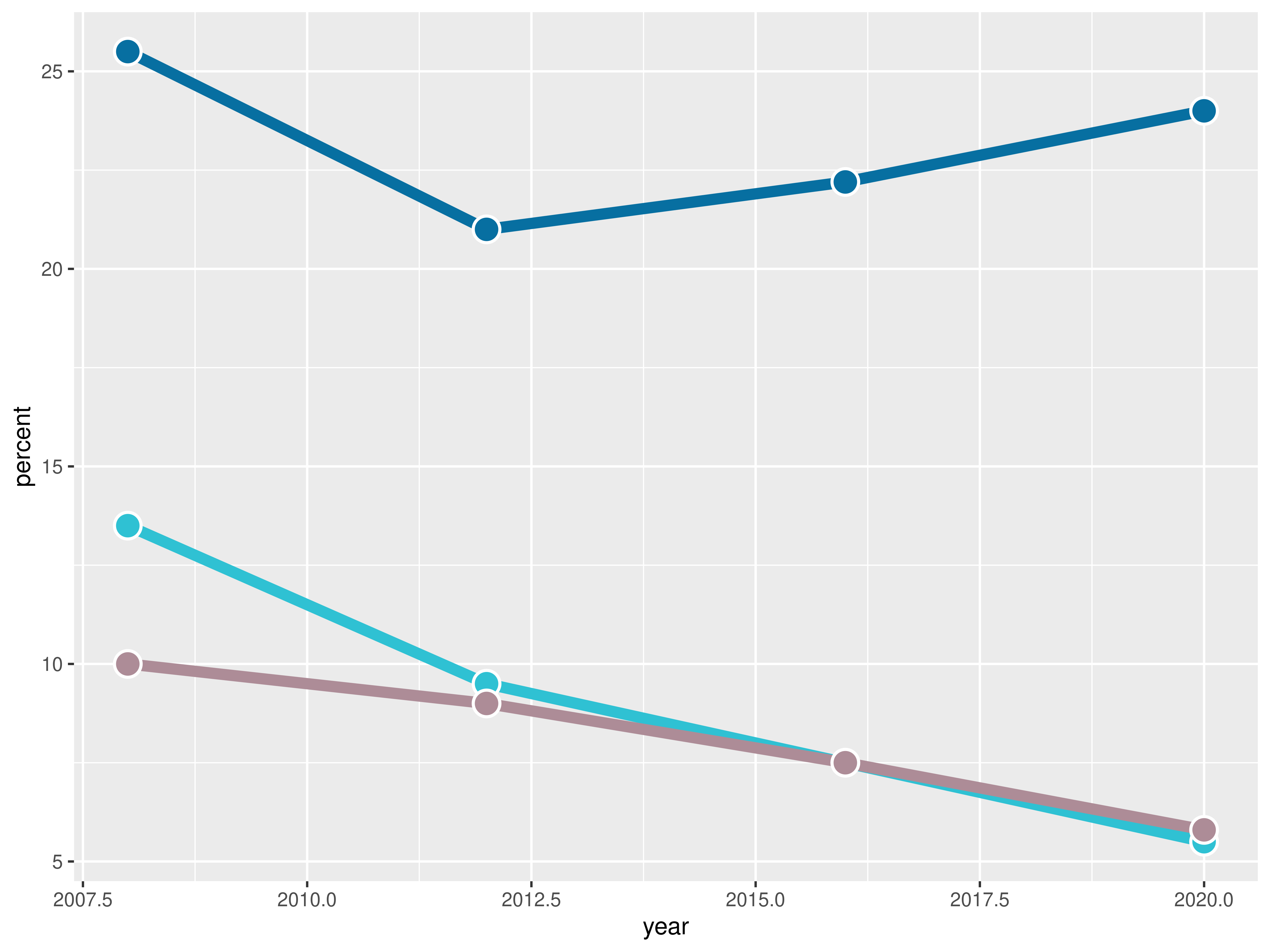It’s been a fair start so far, but there’s still lot to do! Let’s continue with some layout customizations.

# Customize layout

The next step is to customize the layout: change colors, modify axis labels, add grid lines, and many more exciting changes! Let’s do it!

``````plt1 <- plt1 +
scale_x_continuous(
limits = c(2007.5, 2021.5),
expand = c(0, 0), # The horizontal axis does not extend to either side
breaks = c(2008, 2012, 2016, 2020),  # Set custom break locations
labels = c("2008", "12", "16", "20") # And custom labels on those breaks!
) +
scale_y_continuous(
limits = c(0, 32),
breaks = seq(0, 30, by = 5),
expand = c(0, 0)
) +
theme(
# Set background color to white
panel.background = element_rect(fill = "white"),
# Remove all grid lines
panel.grid = element_blank(),
# But add grid lines for the vertical axis, customizing color and size
panel.grid.major.y = element_line(color = "#A8BAC4", size = 0.3),
# Remove tick marks on the vertical axis by setting their length to 0
axis.ticks.length.y = unit(0, "mm"),
# But keep tick marks on horizontal axis
axis.ticks.length.x = unit(2, "mm"),
# Remove the title for both axes
axis.title = element_blank(),
# Only the bottom line of the vertical axis is painted in black
axis.line.x.bottom = element_line(color = "black"),
# Remove labels from the vertical axis
axis.text.y = element_blank(),
# But customize labels for the horizontal axis
axis.text.x = element_text(family = "Econ Sans Cnd", size = 16)
)

plt1``````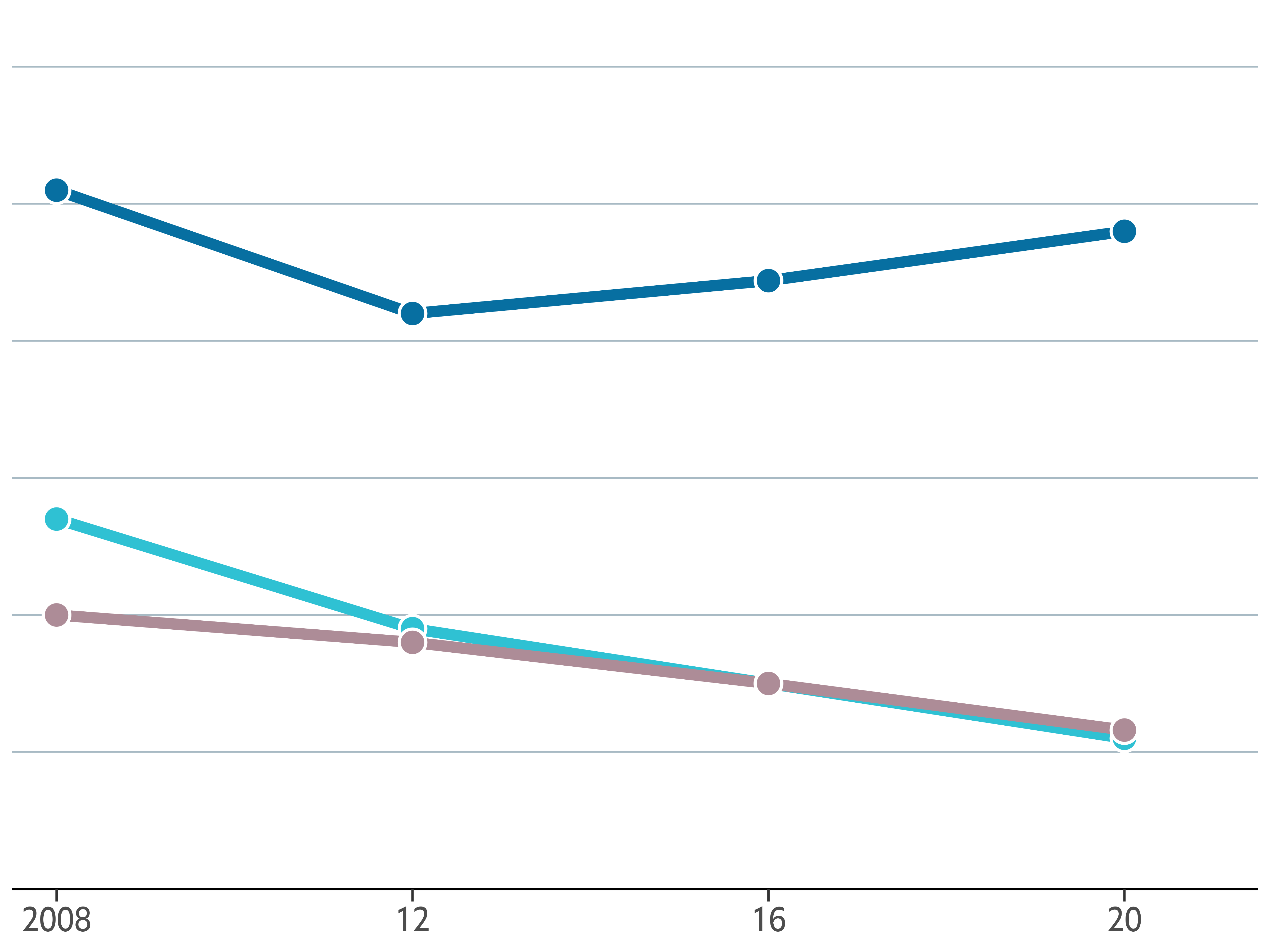It definitely starts to look very nice! 🤩

The chart doesn’t still indicate anything about the regions represented by each line, or the meaning of the vertical axis. It cannot be left like that. This is a good time to improve that!

The following chunk uses both `geom_text()` and `geom_shadowtext()`. The first one is used to draw regular text to indicate the values of the horizontal grid lines that serve as a reference. On the other hand, `geom_shadowtext()` is used to add the labels for the lines. The shadow added covers the horizontal line behind the label for Latin America and the Caribbean region.

In addition, `new_scale_color()` is used to add a new color scale, the one used for the region labels. Although the colors are the same than those added above, `geom_shadowtext()` uses a different data set and thus is considered a new color scale.

Finally, the last step is to add a proper title. Note this title mixes bold and regular text, which is very easy thanks to the `ggtext` package.

``````# Add labels for the lines
plt1 <- plt1 +
new_scale_color() +
aes(x, y, label = labels, color = color),
data = line_labels,
hjust = 0, # Align to the left
bg.colour = "white", # Shadow color (or background color)
bg.r = 0.4, # Radius of the background. The higher the value the bigger the shadow.
family = "Econ Sans Cnd",
size = 6
) +
scale_color_identity() # Use the colors in the 'color' variable as they are.

# Add labels for the horizontal lines
plt1 <- plt1 +
geom_text(
data = data.frame(x = 2021.5, y = seq(0, 30, by = 5)),
aes(x, y, label = y),
hjust = 1, # Align to the right
vjust = 0, # Align to the bottom
nudge_y = 32 * 0.01, # The pad is equal to 1% of the vertical range (32 - 0)
family = "Econ Sans Cnd",
size = 6
)

plt1 <- plt1 +
labs(
title = "**Selected regions,** % of child population",
) +
theme(
# theme_markdown() is provided by ggtext and means the title contains
# Markdown that should be parsed as such (the '**' symbols)
plot.title = element_markdown(
family = "Econ Sans Cnd",
size = 18
)
)

plt1``````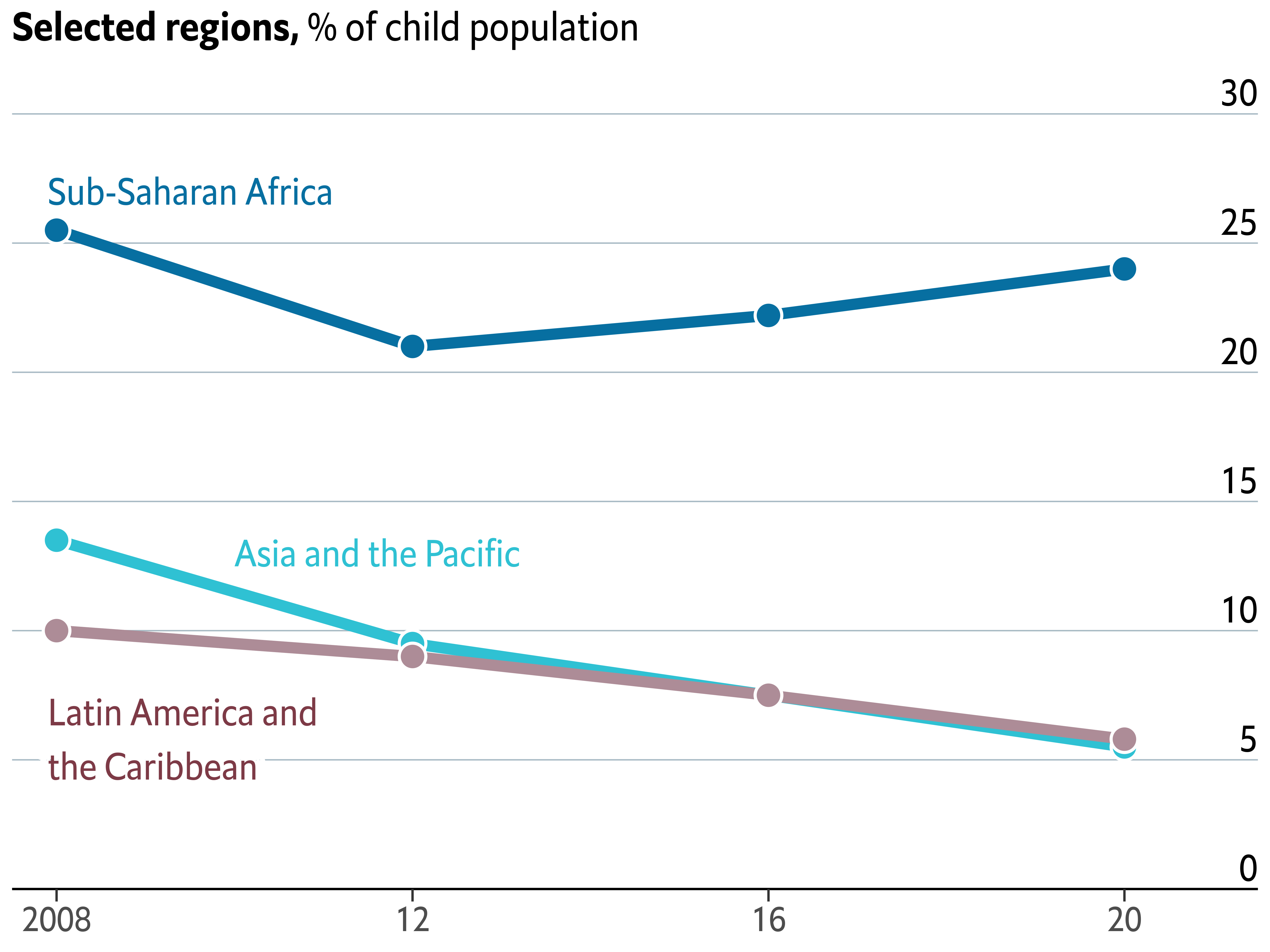# Stacked area chart

Thanks to the `geom_area()` function, it is quite straightforward to create a stacked area chart in `ggplot2`.

``````plt2 <- ggplot(stacked_data) +
# color = "white" indicates the color of the lines between the areas
geom_area(aes(year, percent, fill = region), color = "white") +
scale_fill_manual(values = c(GREY, BROWN, GREEN, BLUE)) +
theme(legend.position = "None") # no legend

plt2``````# Customize layout

As with the previous chart, there’s also a lot to customize here!

``````plt2 <- plt2 +
scale_x_continuous(
# Note: Data goes from 2008 to 2020. Extra space is added on the right
# so there's room for the grid line labels ;)
limits = c(2007.5, 2021.5),
expand = c(0, 0),
breaks = c(2008, 2012, 2016, 2020),
labels = c("2008", "12", "16", "20")
) +
scale_y_continuous(
limits = c(0, 320),
breaks = seq(0, 300, by = 50),
expand = c(0, 0)
) +
theme(
# Set background color to white
panel.background = element_rect(fill = "white"),
# Remove all grid lines
panel.grid = element_blank(),
# But add grid lines for the vertical axis, customizing color and size
panel.grid.major.y = element_line(color = "#A8BAC4", size = 0.3),
# Remove tick marks by setting their length to 0
axis.ticks.length.y = unit(0, "mm"),
axis.ticks.length.x = unit(2, "mm"),
# Remove the title for both axes
axis.title = element_blank(),
# Only bottom line of the vertical axis is painted in black
axis.line.x.bottom = element_line(color = "black"),
# Remove labels from the vertical axis
axis.text.y = element_blank(),
# But customize labels for the horizontal axis
axis.text.x = element_text(family = "Econ Sans Cnd", size = 16)
)

plt2``````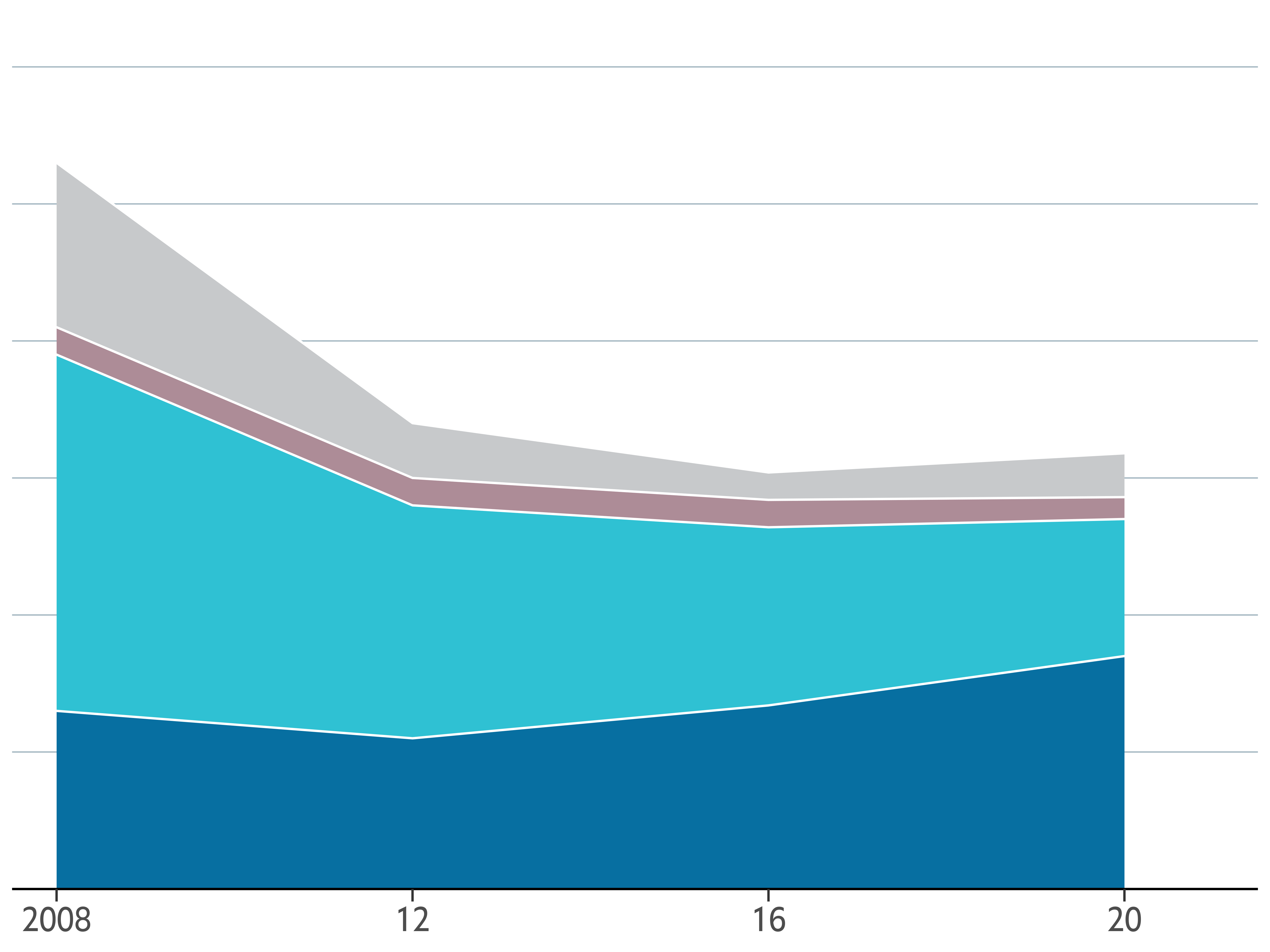We’re about to finish this chart. This last step, which may be the most convoluted step, consists of adding several labels and various annotations. Firs of all, the labels for the areas. Then, the labels for the horizontal gridlines. After that, we make use of the `geom_curve()` function to add a curve that goes from the “Latin America and the Caribbean” label to the area it represents. And finally, it’s time for a title.

Sounds like lot of work? Come on, it’s not going to be that hard!

``````plt2 <- plt2 +
geom_text(
aes(x, y, label = labels, color = color),
data = stacked_labels,
hjust = 0,
vjust = 0.5,
family = "Econ Sans Cnd",
size = 6,
inherit.aes = FALSE
) +
scale_color_identity()

plt2 <- plt2 +
geom_text(
data = data.frame(x = 2021.5, y = seq(0, 300, by = 50)),
aes(x, y, label = y),
hjust = 1,
vjust = 0,
nudge_y = 300 * 0.01, # Again, the pad is equal to 1% of the vertical range.
family = "Econ Sans Cnd",
size = 6,
inherit.aes = FALSE
)

plt2 <- plt2 +
geom_curve(
aes(x = x, y = y, xend = xend, yend = yend),
data = data.frame(x = 2016.9, y = 210, xend = 2018.8, yend = 138),
curvature = -0.5,
angle = 90
) +
geom_point(
aes(x, y),
data = data.frame(x = 2018.8, y = 138),
color = "black"
)

# Note again we use `element_markdown()` to render Markdown content
plt2 <- plt2 +
labs(
title = "**Number of children,** m",
) +
theme(
plot.title = element_markdown(
family = "Econ Sans Cnd",
size = 18
)
)

plt2``````# Combine charts

Now it comes one of the most exciting steps: combining the charts! Thankfully, it exists `patchwork` which makes it extremely easy to combine plots made with `ggplot2`.

The following chunk not only combines the charts, it also adjust their horizontal margins so the result has some space between the charts as in the original figure.

Next, we add a title to the plot obtained with patchwork using `plot_annotation()` and a theme created for it.

``````plt1 <- plt1 + theme(plot.margin = margin(0, 0.05, 0, 0, "npc"))
plt2 <- plt2 + theme(plot.margin = margin(0, 0, 0.05, 0, "npc"))
plt <- plt1 | plt2

title_theme <- theme(
plot.title = element_text(
family = "Econ Sans Cnd",
face = "bold",
size = 22,
margin = margin(0.8, 0, 0, 0, "npc")
),
plot.subtitle = element_text(
family = "Econ Sans Cnd",
size = 20,
margin = margin(0.4, 0, 0, 0, "npc")
)
)

plt <- plt + plot_annotation(
title = "All work, no play",
subtitle = "Children in child labour*",
theme = title_theme
) +
theme(
plot.margin = margin(0.075, 0, 0.1, 0, "npc"),
)

plt``````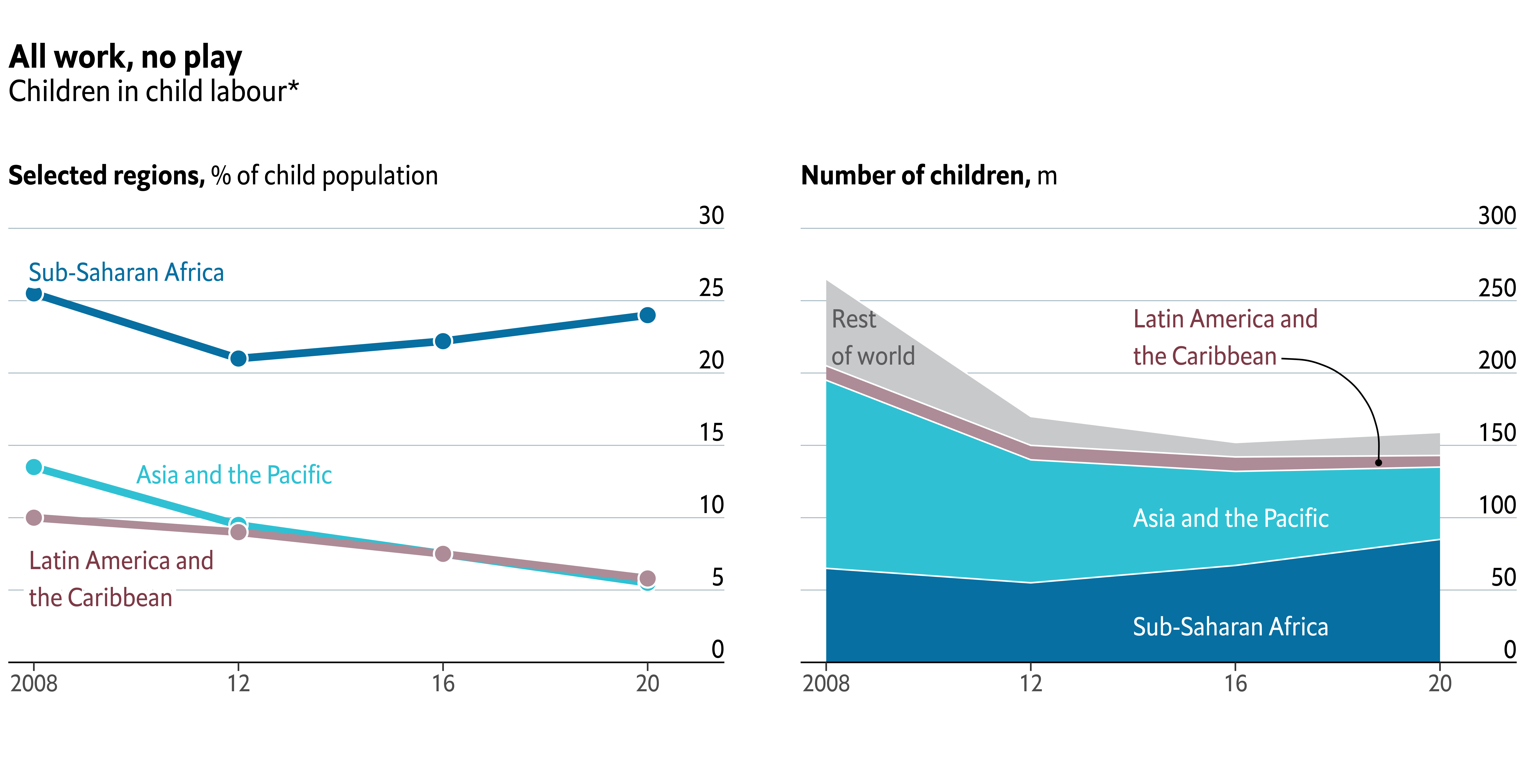It’s so satisfying to see we’re so close! Let’s make one last effort and finish this chart!

# Add final annotations with the `grid` library

The chart above is quite a good replicate of the original figure, but it is clearly missing some small, but very important, details. These are the distinctive red marks on top, and the captions with information about the source of the data and credit to the original author.

We’re going to use the `grid` library for this last task. `grid` is a low-level plotting library that comes with any `R` installation by default and provides many plotting primitive functions. It is also the library that `ggplot2` uses to create the charts under the hood, and that’s why we can combine them in the same chart.

Using `grid` will give us full control of what is added and where it is added to the plot. The downside, is that it requires us to write a considerable amount of extra code.

``````plt

# Add line on top of the chart
grid.lines(
x = c(0, 1),
y = 1,
gp = gpar(col = "#e5001c", lwd = 4)
)

# lwd = 0 means the rectangle does not have an outer line
# 'just' gives the horizontal and vertical justification
grid.rect(
x = 0,
y = 1,
width = 0.05,
height = 0.025,
just = c("left", "top"),
gp = gpar(fill = "#e5001c", lwd = 0)
)
grid.text(
'Source: "Child Labour: Global estimates 2020, trends and the road forward", ILO and UNICEF',
x = 0.005,
y = 0.06,
just = c("left", "bottom"),
gp = gpar(
col = "grey50",
fontsize = 16,
fontfamily = "Econ Sans Cnd"
)
)

grid.text(
"The Economist",
x = 0.005,
y = 0.005,
just = c("left", "bottom"),
gp = gpar(
col = "grey50",
fontsize = 16,
fontfamily = "Milo TE W01"
)
)

grid.text(
"*5- to 17- year-olds",
x = 0.995,
y = 0.06,
just = c("right", "bottom"),
gp = gpar(
col = "grey50",
fontsize = 16,
fontfamily = "Econ Sans Cnd"
)
)``````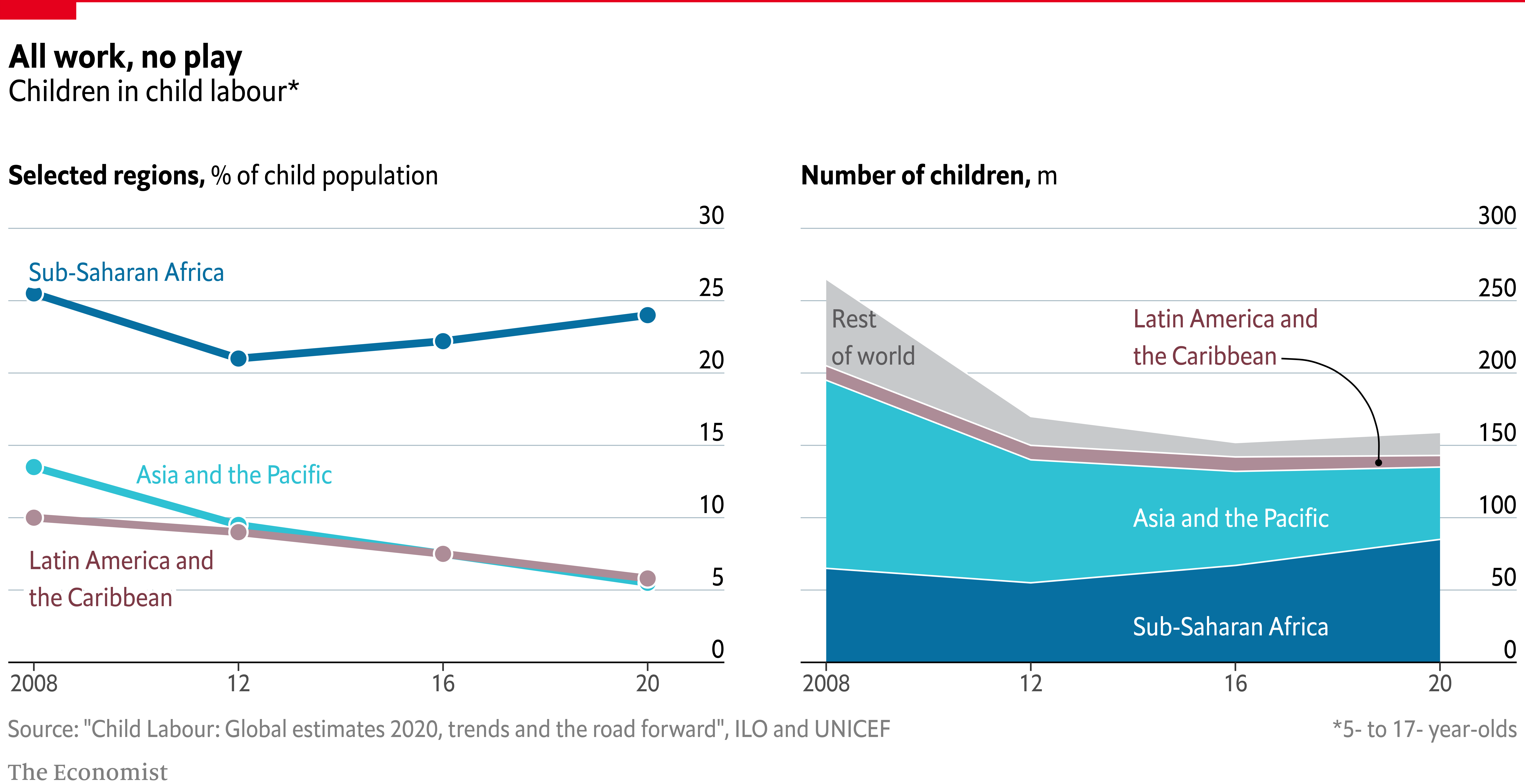Voilà! We nailed it! 🎉

# The extra mile

If you are attentive to the smallest of the details you may have noticed the titles in the chart above aren’t aligned to the leftmost side of the figure as in the original chart.

One alternative, the one we used here, is to remove the titles made with `ggplot2` and make all the annotations from scratch using the `grid` library. Do you like challenges? Then have a look at the article and go for it!

Related chart types

## Contact

This document is a work by Yan Holtz. Any feedback is highly encouraged. You can fill an issue on Github, drop me a message on Twitter, or send an email pasting yan.holtz.data with gmail.com.